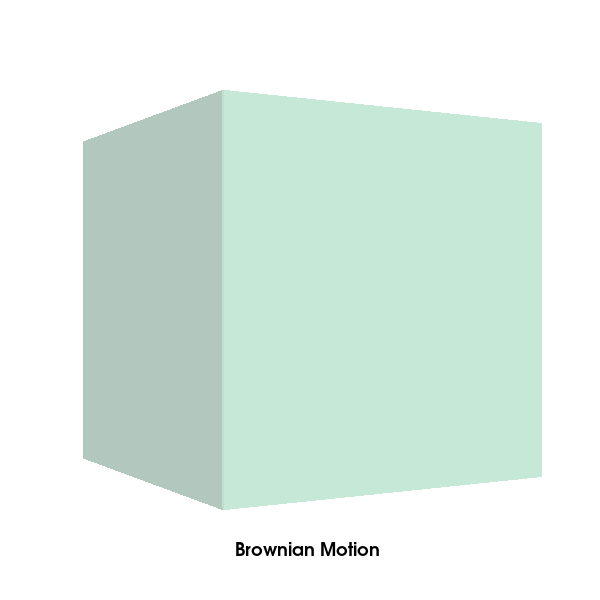# Brownian motion#

Brownian motion, or pedesis, is the random motion of particles suspended in a medium. In this animation, path followed by 20 particles exhibiting brownian motion in 3D is plotted.

Importing necessary modules

```import numpy as np
from scipy.stats import norm

from fury import actor, ui, utils, window
```

Let’s define some variable and their description:

• total_time: time to be discretized via time_steps (default: 5)

• num_total_steps: total number of steps each particle will take (default: 300)

• time_step: By default, it is equal to total_time / num_total_steps

• counter_step: to keep track of number of steps taken (initialised to 0)

• delta: delta determines the “speed” of the Brownian motion. Increase delta to speed up the motion of the particle(s). The random variable of the position has a normal distribution whose mean is the position at counter_step = 0 and whose variance is equal to delta**2*time_step. (default: 1.8)

• num_particles: number of particles whose path will be plotted (default: 20)

• path_thickness: thickness of line(s) that will be used to plot the path(s) of the particle(s) (default: 3)

• origin: coordinate from which the the particle(s) begin the motion (default: [0, 0, 0])

```total_time = 5
num_total_steps = 300
counter_step = 0
delta = 1.8
num_particles = 20
path_thickness = 3
origin = [0, 0, 0]
```

We define a particle function that will return an actor, store and update coordinates of the particles (the path of the particles).

```def particle(
colors,
origin=[0, 0, 0],
num_total_steps=300,
total_time=5,
delta=1.8,
path_thickness=3,
):
origin = np.asarray(origin, dtype=float)
position = np.tile(origin, (num_total_steps, 1))
path_actor = actor.line([position], colors, linewidth=path_thickness)
path_actor.position = position
path_actor.delta = delta
path_actor.num_total_steps = num_total_steps
path_actor.time_step = total_time / num_total_steps
path_actor.vertices = utils.vertices_from_actor(path_actor)
path_actor.no_vertices_per_point = len(path_actor.vertices) / num_total_steps
path_actor.initial_vertices = path_actor.vertices.copy() - np.repeat(
position, path_actor.no_vertices_per_point, axis=0
)
return path_actor
```

The function update_path will simulate the the brownian motion.

```def update_path(act, counter_step):
if counter_step < act.num_total_steps:
x, y, z = act.position[counter_step - 1]
x += norm.rvs(scale=act.delta**2 * act.time_step)
y += norm.rvs(scale=act.delta**2 * act.time_step)
z += norm.rvs(scale=act.delta**2 * act.time_step)
act.position[counter_step:] = [x, y, z]
act.vertices[:] = act.initial_vertices + np.repeat(
act.position, act.no_vertices_per_point, axis=0
)
utils.update_actor(act)
```

Creating a scene object and configuring the camera’s position

```scene = window.Scene()
scene.background((1.0, 1.0, 1.0))
scene.zoom(1.7)
scene.set_camera(
position=(0, 0, 40), focal_point=(0.0, 0.0, 0.0), view_up=(0.0, 0.0, 0.0)
)
showm = window.ShowManager(
scene, size=(600, 600), reset_camera=True, order_transparent=True
)
```

Creating a list of particle objects

```l_particle = [
particle(
colors=np.random.rand(1, 3),
origin=origin,
num_total_steps=num_total_steps,
total_time=total_time,
path_thickness=path_thickness,
)
for _ in range(num_particles)
]

```

Creating a container (cube actor) inside which the particle(s) move around

```container_actor = actor.box(
centers=np.array([[0, 0, 0]]), colors=(0.5, 0.9, 0.7, 0.4), scales=6
)
```

Initializing text box to display the name of the animation

```tb = ui.TextBlock2D(bold=True, position=(235, 40), color=(0, 0, 0))
tb.message = 'Brownian Motion'
```

The path of the particles exhibiting Brownian motion is plotted here

```def timer_callback(_obj, _event):
global counter_step, list_particle
counter_step += 1
for p in l_particle:
update_path(p, counter_step=counter_step)
showm.render()
scene.azimuth(2)
if counter_step == num_total_steps:
showm.exit()
```

Run every 30 milliseconds

```showm.add_timer_callback(True, 30, timer_callback)
showm.start()
window.record(showm.scene, size=(600, 600), out_path='viz_brownian_motion.png')
```Total running time of the script: ( 0 minutes 9.435 seconds)

Gallery generated by Sphinx-Gallery